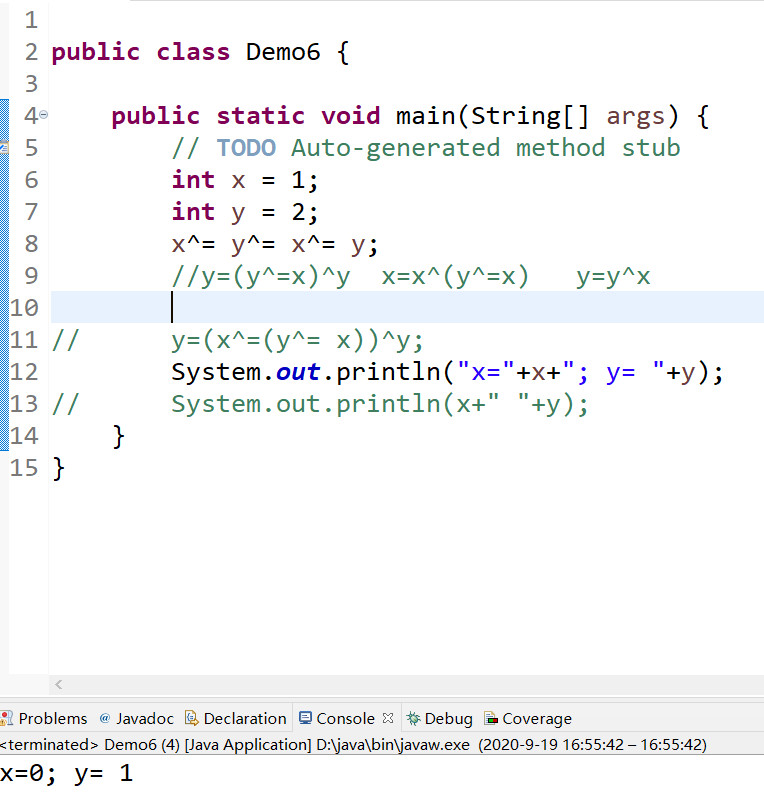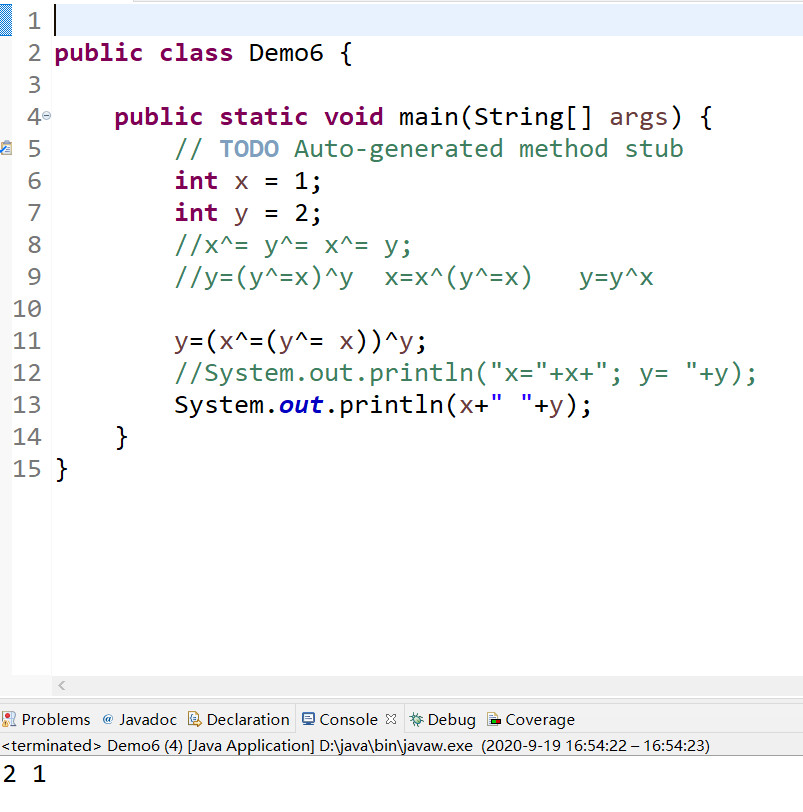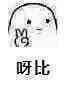# Java-异或运算^

^这个运算符是按位异或运算，就是位运算

x^=y^=y; 这个语句可以看做下面的两条语句

y=y^y;(y和y的值都是一样的  所以按位异或运算后，y=0)

x=x^y;（这里的y已经在上面一条语句中变成0了，x在和y按位异或运算的话  ，值还是x）

```public static void main(String[] args) {
int x = 1984;
int y = 2001;
x^= y^= x^= y;
System.out.println("x="+x+"; y= "+y);
}
//结果y=1984,x=0`````````x = x ^ y;
y = y ^ x;
x = y ^ x;``````
• 1
• 2
• 3

``````        int tmp1 = x;
int tmp2 = y;
int tmp3 = x ^ y;
x = tmp3;
y = tmp2 ^ tmp3;
x = tmp1 ^ y;``````
• 1
• 2
• 3
• 4
• 5
• 6

``y=(x^=(y^=x))^y;``
• 1## Java-简答题

2020-9-19 1:31:43

## Java-选择题

2020-9-19 22:18:10

0 条回复 A文章作者 M管理员
暂无讨论，说说你的看法吧